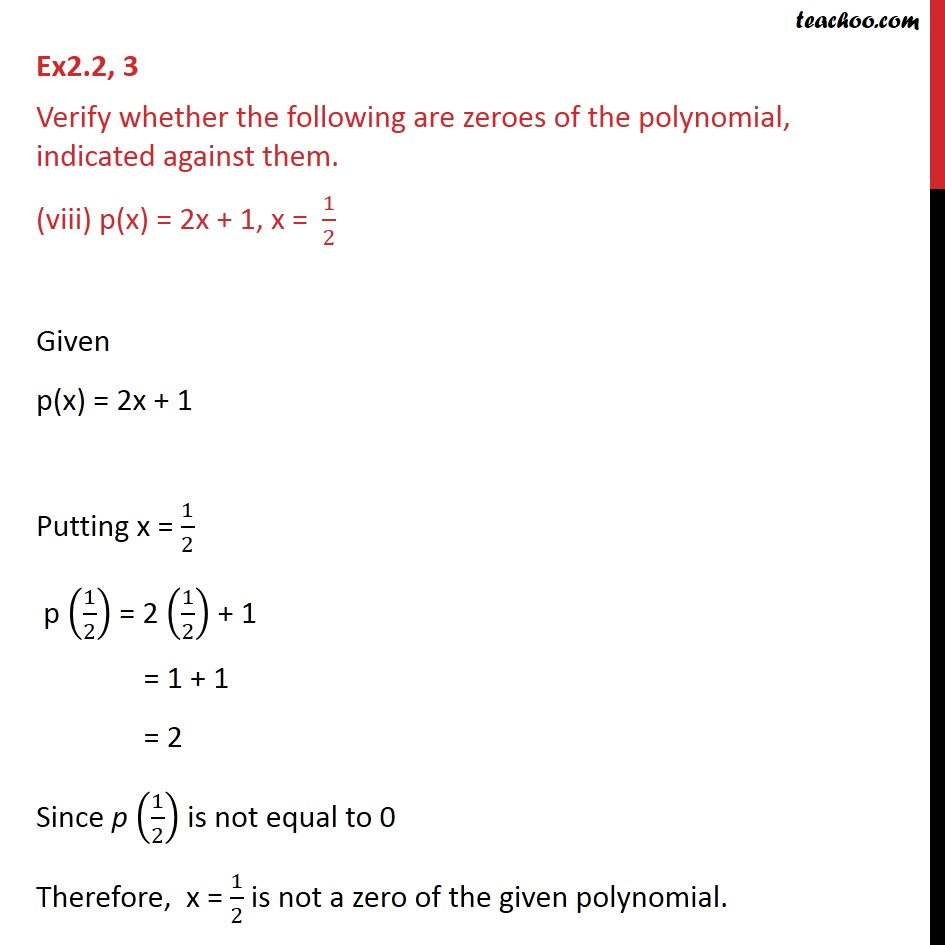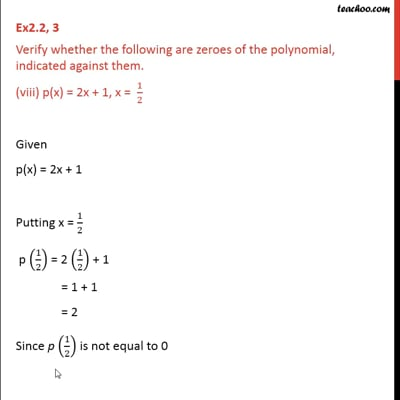Ex 2.2

Chapter 2 Class 9 Polynomials
Serial order wiseThis video is only available for Teachoo black users

Learn in your speed, with individual attention - Teachoo Maths 1-on-1 Class

### Transcript

Ex2.2, 3 Verify whether the following are zeroes of the polynomial, indicated against them. (viii) p(x) = 2x + 1, x = 1/2 Given p(x) = 2x + 1 Putting x = 1/2 p (1/2) = 2 (1/2) + 1 = 1 + 1 = 2 Since p (1/2) is not equal to 0 Therefore, x = 1/2 is not a zero of the given polynomial.